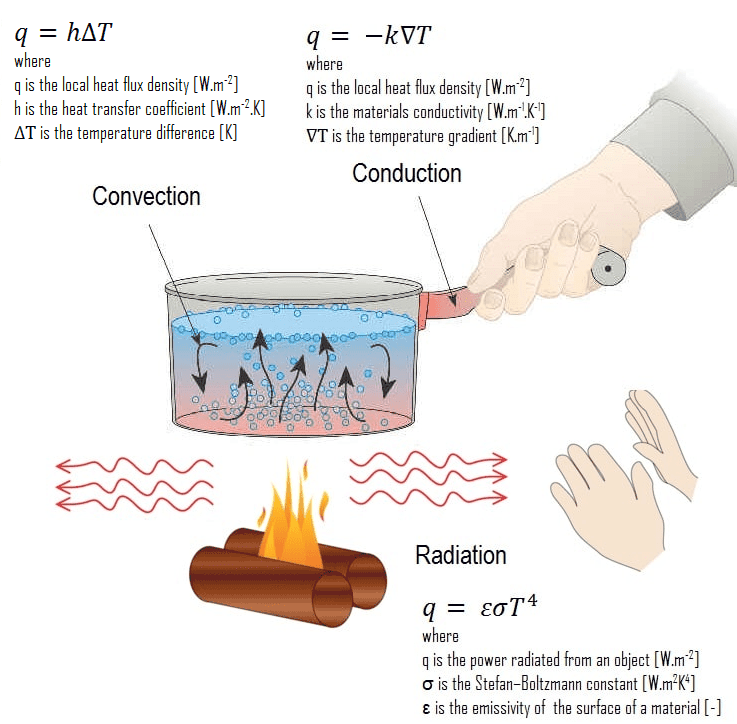# CBSE Class 11 Physics Chapter 11 Revision Notes

Chapter 11: Thermal Properties of Matter Revision Notes

• Heat is a form of energy that is transferred by temperature difference between two (or more) systems or between a system and its surroundings. The joule is the SI unit for heat energy transferred (J).
• Calorie and kilocalorie are the heat units in the CGS system (kcal).

1 kcal = 1000 cal = 4186 J, and 1 cal = 4.186 J.

• The temperature of a substance is a physical quantity that indicates how hot or cold the substance is. The kelvin (K) is the SI unit of temperature, and the degree Celsius (°C) is a commonly used unit of temperature.
• Thermometry is a branch of science concerned with the measurement of a substance’s temperature. A thermometer is a device that measures a person’s body temperature.
• The value of a given temperature on a temperature scale is measured using a thermometer calibrated for that scale. Two fixed reference points are chosen for temperature measurement. The ice point and steam point of water at standard pressure, also known as the freezing point and boiling point of water at standard pressure, are two useful fixed reference points.
• The Fahrenheit temperature scale and the Celsius temperature scale are two well-known temperature scales. On the Fahrenheit scale, the ice and steam points are 32°F and 212°F, respectively, and on the Celsius scale, 0°C and 100°C. There are 180 equal intervals between two reference points on the Fahrenheit scale, and 100 on the Celsius scale.
• If tc and tF are the temperature values of a body on the Celsius and Fahrenheit temperature scales, respectively.
• The absolute minimum temperature for an ideal gas is – 273.15 °C, which is designated as absolute zero by extrapolating the straight line P – T graph. t° C = T – 273.15 is the relationship between the absolute temperature scale (T) and the Celsius scale (C).

# Expansion due to Heat

• The term “thermal expansion” refers to the expansion of a body as the temperature rises. In solids, there are three types of expansions: linear, superficial, and volume expansion.1. Linear Expansion: Linear expansion refers to the increase in the length of a solid as a result of heating.

• When the temperature of a rod with an original length of l is raised by a small amount Δt, the length of the rod increases by Δl. The linear expansion is then given by

Δl = l ∞ Δt

2. Superficial or Area Expansion: Superficial expansion refers to the increase in the solid’s surface area as a result of heating.

3. Volume Expansion: The term “volume expansion” refers to the increase in volume of a solid as a result of heating.

• The change in volume of a solid as a function of temperature Δt is given by

Δv = Vγ Δt, where y is the volume expansion coefficient.

• Thermal Stress: When the temperature of a rod held between two fixed supports rises, the fixed supports prevent the rod from expanding, resulting in a stress known as thermal stress.

• Thermal Capacity: The amount of heat required to raise the temperature of the entire body by one degree is the thermal capacity of a body. Calorie per °C or joule per K are the units of measurement.

• Specific Heat Capacity: A substance’s specific heat capacity (also known as specific heat) is the amount of heat required to raise the temperature of a unit mass of substance by one degree Celsius. Js-1 m-1 °C-1 or W-1 K-1 are the units of measurement.

• Calorimetry: Calorimetry is concerned with the measurement of heat, and the calorimeter is the most basic apparatus for this purpose.

• When two bodies of different temperatures are mixed, heat flows from one to the other until a common ‘equilibrium’ temperature is reached. Assuming that this ‘heat exchange’ is limited to the two bodies (i.e., no heat loss to the environment), we can deduce from the law of energy conservation that: Heat gained by one body = heat lost by the other.

• A change of state is the transition of matter from one state (solid, liquid, or gas) to another.

• Melting is the change of state from solid to liquid, and fusion is the change of state from liquid to solid. The temperature remains constant until the entire amount of solid substance melts, indicating that both the solid and liquid states of the substance co-exist in thermal equilibrium during the transition from solid to liquid.

• The melting point of a solid is the temperature at which it melts. The melting point of a solid is a property of the substance and is also affected by pressure.

• Regulation is the melting of ice under increased pressure and refreezing when the pressure is reduced; vaporisation is the change of state from liquid to vapour (or gas). The temperature at which a substance’s liquid and vapour states coexist is known as the boiling point.

• Sublimation is the transition from a solid to a gaseous state without passing through a liquid state.

## Transfer of Heat

Conduction, convection, and radiation are three methods for transferring heat from one location to another. Conduction occurs in solids, convection in liquids and gases, and radiation does not require a medium.Source

(i) Conduction: According to Maxwell, conduction is the flow of heat from places of higher temperature to places of lower temperature through an unequally heated body.

(ii) Convection: According to Maxwell, convection is the flow of heat caused by the hot body’s motion, which carries the heat with it.

(iii) Radiation: Radiation is a type of heat transfer in which heat is transferred directly from one location to another without the use of a medium in between.

]]>